# Tesla Debt Leverage And Capital Structure Analysis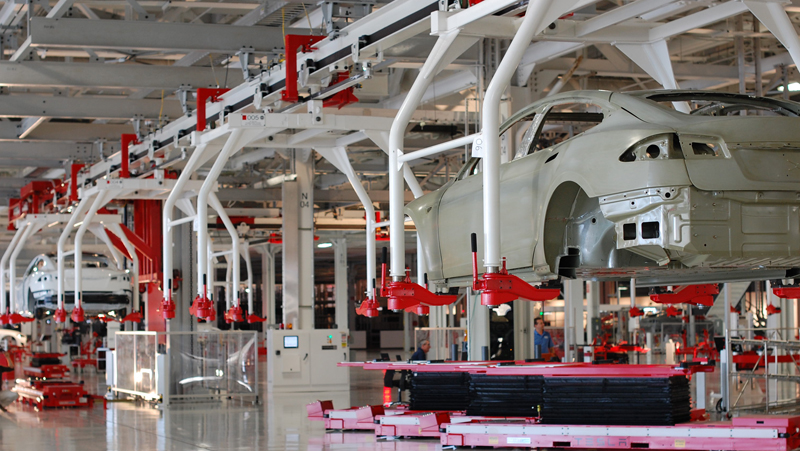A Tesla factory in Fremont, CA. Source: Flickr

Tesla (NASDAQ: TSLA) debt to equity ratio measures the debt leverage of the company with respect to equity.

The equity portion is the net worth or book value of Tesla.

The more debt Tesla has, the higher the debt-to-equity ratio is.

For example, if Tesla has \$1 dollars of equity and \$10 dollars of debt, its debt-to-equity ratio will be 10 to 1 or 10X, meaning that Tesla is leveraging 10X higher than its net worth.

In fact, Tesla is highly leveraged in this case if its debt-to-equity ratio amounts to 10X.

In addition to debt leverage, the debt-to-equity ratio also tells us about the capital structure of Tesla.

For example, we can find out how Tesla funded its balance sheets through the debt-to-asset ratio, whether entirely by debts or by equity, or equally both.

Ideally, the capital structure of a company should be well-balanced between debts and equity.

For example, for every 1 dollar of equity, there is 1 dollar of debt. In this case, the debt-to-equity ratio will be 1.

However, in real life, this balanced debt structure scenario is not always the case for most companies.

The reason is that it may not be prudent to rely largely on equity finances.

In most cases, the cost of debt may be much cheaper than the required rate of return on equity.

This is particularly true in a low-interest-rate environment.

In this article, we will explore Tesla’s debt leverage and capital structure to find out how far the company has leveraged and funded its expansion, whether by debts or by equity.

To do this, we will use ratios such as the debt-to-equity ratio and debt-to-asset ratio.

Let’s get started!

## Equation For Debt To Equity Ratio

Before illustrating further, the equation that I used to calculate the debt-to-equity ratio is shown below:

Debt To Equity Ratio = Debt / Equity

From the above equation, the equity part is easily understood.

Basically, it’s the total equity or the book value that can be easily obtained from the balance sheets.

## Tesla’s Equity

Tesla’s equities include both shareholders’ equity and minority interest equity.

Two types of equities exist because Tesla is a holding company of multiple subsidiaries.

As such, the balance sheet is consolidated with the assets and liabilities of all subsidiaries.

The consolidation has led to the existence of minority interests in the balance sheet since Tesla does not own 100% of some subsidiaries.

The following snapshot illustrates Tesla’s non-controlling interest or minority interest portion in the balance sheet.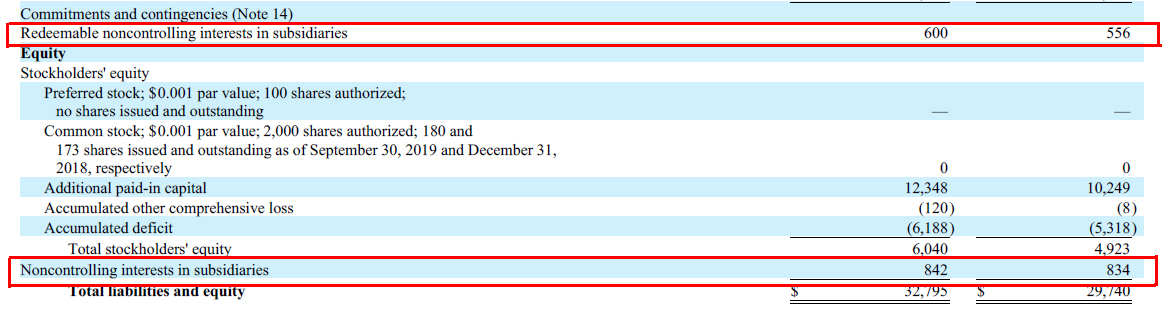Tesla minority interest

Tesla’s minority interest as shown in the balance sheet above is due to the company’s ownership structure.

## Tesla’s Debt Definitions

From the debt-to-equity ratio, difficulties may arise when we try to figure out the debt part of the equation.

There are countless definitions of “debts”. In this case, debts can be derived from long-term or current debts, total liabilities, or interest-bearing only debts.

For simplicity, I have considered only the “debts” that matter most in the analysis of Tesla’s leverages.

The following items show the definition of debt in Tesla’s case:

1. Total debts – debt instruments that include both long and short-term loans as well as finance and operating leases. Basically, total debts are all interest-bearing debt instruments.

2. Long-term liabilities – these are long-term loans and other miscellaneous liabilities such as accounts payable, deferred revenues, leases, warranty reserves, and so on. Only the long-term portion is measured in this case.

3. Total liabilities – these are all the liabilities in the balance sheet that are comprised of both long and short-term loans as well as other long and short liabilities such as accounts payable, deferred revenues, and other miscellaneous liabilities.

The subsequent debt-to-equity ratio discussion will revolve around these 3 types of “debt” definitions mentioned above.

## Tesla’s Total Debt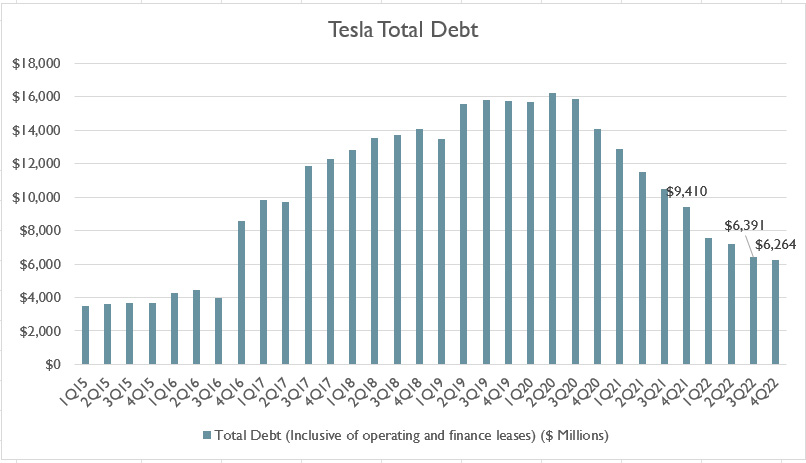Tesla debt and leases

* Total debt figures come from the author’s own calculation and consist of long and short-term debt instruments as well as leases.
* Tesla’s fiscal year begins on Jan 1 and ends on Dec 31.

Let’s first briefly look at Tesla’s total debt as shown in the chart above.

As briefly mentioned in the prior discussion, Tesla’s total debt consists of mainly interest-bearing debt instruments, including long-term and current loans as well as operating and finance leases.

As of 2022 Q4, Tesla’s total debt (inclusive of operating and finance leases) reached a massive \$6.3 billion, representing a decline of 33% compared to the same quarter a year ago.

For your information, finance and operating leases are interest-bearing debt instruments.

In fact, they have added substantial indebtedness to the company as shown in this article: Tesla debts and leases.

The leases, both operating and finance, are the total minimum lease payments or MLPs which also include the interest portion.

The following snapshot shows an example of Tesla’s minimum lease payments disclosed in one of its financial statements: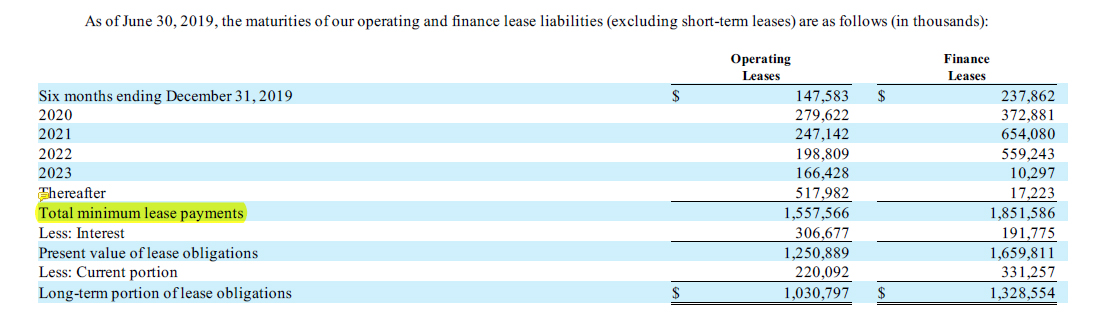Tesla operating and finance leases from the quarterly statement

However, Tesla’s total debt does not include such liabilities as accounts payable, accrued liabilities, deferred revenue, warranty reserves, and so on.

In short, Tesla’s total debt consists of only interest-bearing debt instruments and leases, both finance and operating, and has been on a decline since fiscal 2020.

As of 4Q 2022, this figure arrived at roughly \$6.3 billion USD, a number that was last seen in 2016 when Tesla was racking up its debt.

## Tesla’s Total Debt To Equity Ratio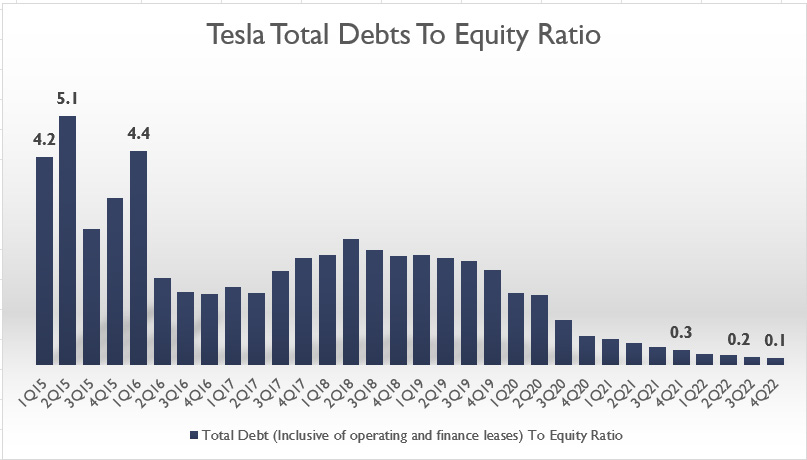Tesla debt to equity ratio

* Debt to equity ratio is calculated by the author based on Tesla’s total debt and equity obtained from the company’s balance sheets.
* Total debt consists of long and short-term debt as well as operating and finance leases.
* Tesla’s fiscal year begins on Jan 1 and ends on Dec 31.

The chart above shows Tesla’s total debts to equity ratio for the period from fiscal 2015 to 2022.

As seen from the chart, Tesla’s total debt-to-equity ratio was the highest back in 2015 when the ratio averaged around 4.0X.

At this ratio, Tesla’s leverage was \$4 dollars of debt to \$1 dollars of equity, illustrating the moderate leverage with respect to equity.

Over the years, Tesla’s debt-to-equity ratio has been declining and reached only 0.1X as of 2022 4Q.

At this ratio, Tesla’s leverage was low as its debt was far less than its equity.

There are a few reasons for Tesla’s declining debt leverage.

First, Tesla has paid off most of its outstanding debt.

Also, Tesla has taken on fewer borrowings to fund its expansion in recent years and thus, the lower debt-to-equity ratio.

Secondly, Tesla has issued more equity in recent years to fund its expansion.

This approach makes sense as Tesla’s share price has been surging to new highs.

In this case, Tesla can issue less equity to obtain the same amount of capital.

Also, equity is less risky compared to debt because Tesla does not have to repay the capital obtained through equity.

The only downside to equity is that it dilutes the control of the company.

For debt, Tesla needs to pay not only the principal but also the interest portion.

However, the advantage of debt is that it’s tax-deductible!

## Tesla Long-Term Liabilities To Equity RatioTesla long-term liabilities to equity ratio

* Long-term liabilities to equity ratio is calculated by the author based on Tesla’s long-term liabilities and equity obtained from the company’s balance sheets.
* Long-term liabilities consist of all long-term debt and other liabilities such as pension funds, taxes due, etc.
* Tesla’s fiscal year begins on Jan 1 and ends on Dec 31.

In terms of long-term liabilities, Tesla’s long-term liabilities to equity ratio plot looks very similar to the total debts to equity ratio plot which we saw earlier.

In fact, the trend of the long-term liabilities to equity ratio plot looks exactly the same as the prior chart.

For instance, Tesla was highly leveraged back in 2015 when the long-term liabilities to equity ratio averaged around 3.8X.

Over the years, the ratio has significantly declined and reached only 0.2X as of Q4 2022.

At this ratio, Tesla was minimally leveraged at \$0.20 dollar of long-term liabilities to \$1.00 dollar of equity.

Similarly, Tesla’s declining total liabilities to equity ratio can be attributed to the declining debt and decreasing indebtedness of the company in recent years.

Additionally, Tesla’s surging stock price has caused equity issuance to be less risky and cheaper compared to debt borrowings.

In this case, Tesla can issue far fewer equities to obtain a larger capital.

## Tesla Total Liabilities To Equity RatioTesla total liabilities to equity ratio

* Total liabilities to equity ratio is calculated by the author based on Tesla’s total liabilities and equity obtained from the company’s balance sheets.
* Total liabilities consist of all long-term and current debt and other liabilities such as accounts payable, accrued liabilities, pension funds, taxes due, etc.
* Tesla’s fiscal year begins on Jan 1 and ends on Dec 31.

The chart above shows Tesla’s total liabilities to equity ratio for the past 6 years from fiscal 2015 to 2022.

Tesla’s total liabilities are basically all long and short-term borrowings and whatever the company owes to 3rd parties in the balance sheets.

In terms of total liabilities, the trend of Tesla’s total liabilities to equity ratio also looks similar to prior charts.

The only difference is that the leverage ratio has been much higher in the current chart.

This is expected as all liabilities are included in the measurement of the debt-to-equity ratio.

Again, a similar trend is observed for Tesla’s total liabilities to equity ratio.

In this case, Tesla used to have a higher ratio at more than 6.0X in fiscal 2015.

However, this ratio has since declined and reached only 0.8X as of 4Q 2022, the lowest figure the company has ever seen.

At this ratio, Tesla has a balanced debt structure at \$0.80 dollars of total liabilities to \$1.00 dollars of equity.

Again, the 0.8X ratio shows that Tesla is moderately leveraged and the company should be doing fine with this level of liabilities.

Tesla has been getting rid of debt and has been inclined to take on more debt in recent quarters and thereby leading to the record low debt-to-equity ratio.

Instead, Tesla has preferred to finance its expansion through equity issuance since 2020.

The reason is that Tesla’s valuation has been rising steadily and has surged significantly in recent quarters.

Therefore, it is to Tesla’s advantage to obtain capital through equity issuance since the company can issue less equity but is able to get a much larger capital.

Again, Tesla does not have to pay back the capital obtained through equity issuance.

## Tesla’s Capital StructureTesla total liabilities to asset ratio

* Total liabilities to asset ratio is calculated by the author based on Tesla’s total liabilities and total assets obtained from the company’s balance sheets.
* Tesla’s fiscal year begins on Jan 1 and ends on Dec 31.

The equation to calculate the total liabilities to total asset ratio is shown below:

Total Liabilities To Asset Ratio = [ Total Liabilities / Total Assets ] X 100%

The total liabilities to total assets ratio shows Tesla’s capital structure.

As shown in the chart above, Tesla’s capital structure was mostly debt-based or liabilities-based prior to fiscal 2020.

For example, Tesla’s total liabilities to total asset ratio was around 80% between fiscal 2015 and 2019, indicating a much larger portion of liabilities with respect to equity.

However, the ratio has significantly declined in recent quarters and reached only 44% as of 2022 Q4, a record low for Tesla.

At this ratio, Tesla’s capital structure was only 44% of liabilities and 56% of equity.

Tesla was having a balanced capital structure at 45% of liabilities and 55% of equity in 4Q 2022.

Tesla’s capital structure was more on debt or liabilities prior to fiscal 2020 at 80% of liabilities and 20% of equity.

However, this scenario has changed as of fiscal 2022.

When Tesla’s share price surged in 2020, Tesla slowly restructured its capital to an equity-based rather than a debt-based as seen in the declining plot in the chart above.

At this debt composition, Tesla’s leverage was moderately low at about \$0.44 dollars of liabilities to \$1.00 dollars of assets as of Q4 2022.

## Tesla’s Debt To Asset RatioTesla total debt to asset ratio

* Total debt-to-asset ratio is calculated by the author based on Tesla’s total debt and total assets obtained from the company’s balance sheets.
* Tesla’s fiscal year begins on Jan 1 and ends on Dec 31.

Another ratio worth taking a look at is the total debt-to-asset ratio which is shown in the chart above.

Similarly, this ratio also shows Tesla’s debt structure or capital structure.

As shown in the chart above, Tesla used to have a debt-to-asset ratio that averaged around 45% prior to fiscal 2020.

However, this figure has since declined and dropped to only 7.6% as of Q4 2022.

At this ratio, Tesla’s capital structure was less than 10% debt and the rest was equity as well as other liabilities.

Again, the declining ratio reinforces the idea that Tesla has shifted from debt-fueled financing to equity-fueled financing.

Tesla was minimally leveraged based on this ratio and has only \$0.07 dollars of debt to \$1.00 dollars of assets.

In short, Tesla uses less debt now and preferred equity-based financing to fund its expansion due primarily to the surging stock price in recent quarters.

## Conclusion

To recap, Tesla’s total debts to equity ratio has significantly declined from its peak value of more than 4.0X recorded in fiscal 2015 to only 0.1X as of 2022 Q4.

The downtrend is also observed for the total liabilities to equity ratio, indicating that Tesla’s preference for funding has shifted towards equity over debt.

The trend is expected when Tesla’s valuation is slowly gaining higher and surpassed \$500 billion as of 4Q 2022.

At this enormous valuation, Tesla can obtain a higher capital through equity issuance even though this practice may mean further stock dilution to its existing stockholders.

Nevertheless, as of 4Q 2022, Tesla is minimally leveraged in terms of debt and has a balanced capital structure at 44% of liabilities and 56% of equity.

## References and Credits

1. Financial figures in all charts above were obtained and referenced from Tesla’s annual and quarterly statements available in Tesla Quarterly Results.

2. Featured image was used under Creative Common Licenses and obtained from mangopulp2008 and Maurizio Pesce.

## Disclosure

If you find the information in this article helpful, please consider sharing it on social media and also provide a link back to this article from any website so that more articles like this one can be created in the future.

Thank you!

## Related Statistics For Other Stocks

•Anna December 18, 2020, 7:21 am

Hello!
Just wondering how did you calculate total debt to equity ratio as I am slightly confused looking at tesla’s financial statements. Thank you!

•cckean December 18, 2020, 9:42 am

Just take the total debt and divide it over the total equity.